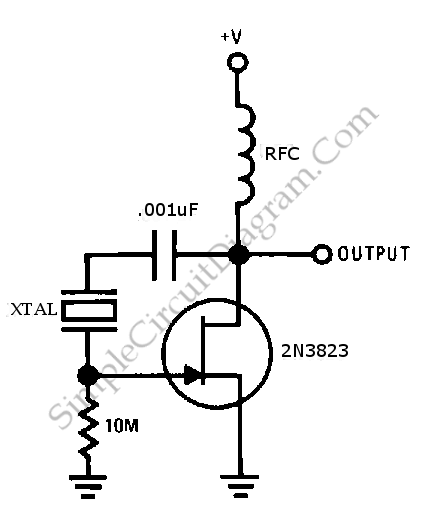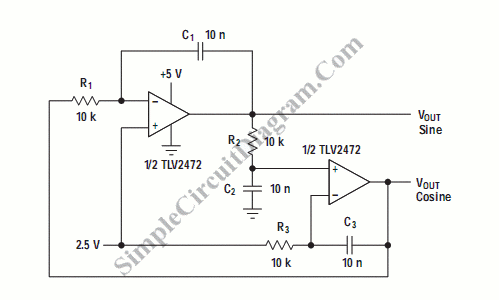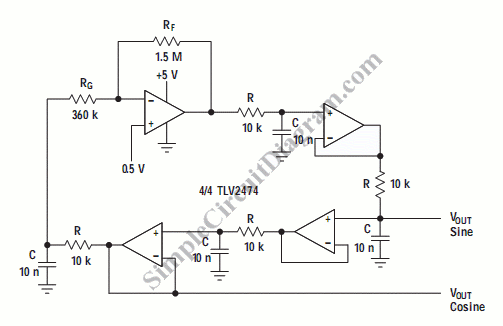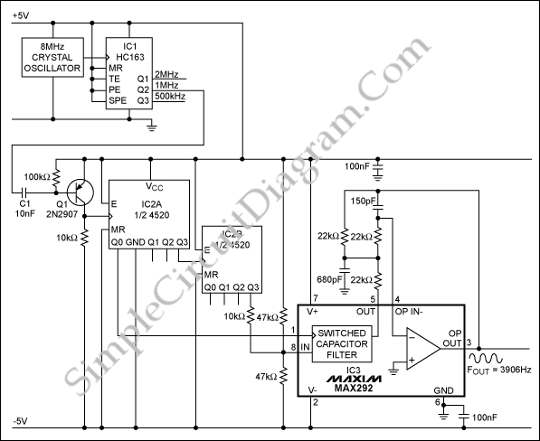## JFET Pierce Crystal OscillatorThis is a simple JFET pierce crystal oscillator. We can use a wide frequency range of crystal using this circuit without circuit modification. Here is the circuit : This circuit uses Q, 2N3823, that is maintained thus insuring good stability of frequency because the crystal does not loaded by the JFET. [Source: National Semiconductor Application Note]Quadrature oscillator is another type of phase shift oscillator. This circuit produce two sine wave signal. One of them is shifted 90 degree from the other signal. The output that shifted 90 degree is called cosine signal and the other is called sine circuit has two output. Here is the circuit : This circuit has three phase shifter sections. Each […]

## Bubba OscillatorOne example of the  phase shift oscillator is Bubba oscillator. This oscillator gets 45 degree phase shift for each section from quad op-amp package. The result is excellent d(phase)/d(t) to obtain very low frequency drift. The circuit is shown below: 45 degree phase shift is obtained from Each RC section. The alternating section generate a low impedance quadrature output. The […]